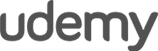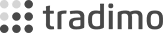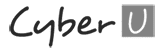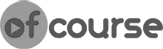# Moving Average Filter – MQL4 for Complete Beginners Tutorial Part 29

In this tutorial we will add the moving average indicator as an additional market entry condition to our trading strategy. To do this we will use the iMA() function which is built-in into MQL4. Functions such as iMA, iMACD, iRSI and many others allow you to quickly access the Moving Average, MACD, RSI and other indicators from within your Algorithmic Trading Systems. In this beginners course we will only touch on the iMA() briefly, but that should be enough for what we need in our trading system.

Enrol in the full course here: https://www.forexboat.com/learn-mql4

## Code for 4-digit brokers

```//+------------------------------------------------------------------+ //| SimpleSystem.mq4 | //| Copyright 2014, ForexBoat | //| http://www.forexboat.com | //+------------------------------------------------------------------+ #property copyright "Copyright 2014, ForexBoat" #property link "http://www.forexboat.com" #property version "1.00" #property strict```

``` extern int StartHour = 9; extern int TakeProfit = 40; extern int StopLoss = 40; extern double Lots = 1; extern int MA_Period = 100; void OnTick() { static bool IsFirstTick = true; static int ticket = 0; double ma = iMA(Symbol(), Period(), MA_Period, 0, 0, 0, 1); if(Hour() == StartHour) { if(IsFirstTick == true) { IsFirstTick = false; bool res; res = OrderSelect(ticket, SELECT_BY_TICKET); if(res == true) { if(OrderCloseTime() == 0) { bool res2; res2 = OrderClose(ticket, Lots, OrderClosePrice(), 10); if(res2 == false) { Alert("Error Closing Order #", ticket); } } } if(Open < Open[StartHour]) { //check ma if(Close < ma) { ticket = OrderSend(Symbol(), OP_BUY, Lots, Ask, 10, Bid-StopLoss*Point, Bid+TakeProfit*Point, "Set by SimpleSystem"); if(ticket < 0) { Alert("Error Sending Order!"); } } } else { //check ma if(Close > ma) { ticket = OrderSend(Symbol(), OP_SELL, Lots, Bid, 10, Ask+StopLoss*Point, Ask-TakeProfit*Point, "Set by SimpleSystem"); if(ticket < 0) { Alert("Error Sending Order!"); } } } } } else { IsFirstTick = true; } ```

```} ```

## Code for 5-digit brokers

```//+------------------------------------------------------------------+ //| SimpleSystem.mq4 | //| Copyright 2014, ForexBoat | //| http://www.forexboat.com | //+------------------------------------------------------------------+ #property copyright "Copyright 2014, ForexBoat" #property link "http://www.forexboat.com" #property version "1.00" #property strict```

``` extern int StartHour = 9; extern int TakeProfit = 40; extern int StopLoss = 40; extern double Lots = 1; extern int MA_Period = 100; void OnTick() { static bool IsFirstTick = true; static int ticket = 0; double ma = iMA(Symbol(), Period(), MA_Period, 0, 0, 0, 1); if(Hour() == StartHour) { if(IsFirstTick == true) { IsFirstTick = false; bool res; res = OrderSelect(ticket, SELECT_BY_TICKET); if(res == true) { if(OrderCloseTime() == 0) { bool res2; res2 = OrderClose(ticket, Lots, OrderClosePrice(), 10*10); if(res2 == false) { Alert("Error Closing Order #", ticket); } } } if(Open < Open[StartHour]) { //check ma if(Close < ma) { //here we are assuming that the TakeProfit and StopLoss are entered in Pips ticket = OrderSend(Symbol(), OP_BUY, Lots, Ask, 10*10, Bid-StopLoss*Point*10, Bid+TakeProfit*Point*10, "Set by SimpleSystem"); if(ticket < 0) { Alert("Error Sending Order!"); } } } else { //check ma if(Close > ma) { //here we are assuming that the TakeProfit and StopLoss are entered in Pips ticket = OrderSend(Symbol(), OP_SELL, Lots, Bid, 10*10, Ask+StopLoss*Point*10, Ask-TakeProfit*Point*10, "Set by SimpleSystem"); if(ticket < 0) { Alert("Error Sending Order!"); } } } } } else { IsFirstTick = true; } ```

`}`

What are you waiting for?

## START LEARNING FOREX TODAY!•••••